Next: Worked Examples Up: Electricity Previous: Coulomb's Law

## Electric Fields

According to Coulomb's law, a chargeexerts a force on a second charge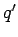, and vice versa, even in a vacuum. But, how is this force transmitted through empty space? In order to answer this question, physicists in the 19th Century developed the concept of an electric field. The idea is as follows. The chargegenerates an electric fieldwhich fills space. The electrostatic force exerted on the second chargeis actually produced locally by the electric field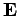at the position of this charge, in accordance with Coulomb's law. Likewise, the chargegenerates its own electric fieldwhich also fills space. The equal and opposite reaction force exerted onis produced locally by the electric fieldat the position of this charge, again, in accordance with Coulomb's law. Of course, an electric field cannot exert a force on the charge which generates it, in just the same way as we cannot pick ourselves up with our own shoelaces. Incidentally, electric fields have a real physical existence, and are not just theoretical constructs invented by physicists to get around the problem of the transmission of electrostatic forces through vacuums. We can say this with certainty because, as we shall see later, there is an energy associated with an electric field filling space. Indeed, it is actually possible to convert this energy into heat or work, and vice versa.

The electric fieldgenerated by a set of fixed electric charges is a vector field which is defined as follows. If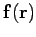is the electrostatic force experienced by some small positive test chargelocated at a certain pointin space, then the electric field at this point is simply the force divided by the magnitude of the test charge. In other words,(62)

Electric field has dimensions of force per unit charge, and units of newtons per coulomb (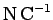). Incidentally, the reason that we specify a small, rather than a large, test charge is to avoid disturbing any of the fixed charges which generate the electric field.

Let us use the above rule to reconstruct the electric field generated by a point charge. According to Coulomb's law, the electrostatic force exerted by a point chargeon a positive test charge, located a distancefrom it, has the magnitude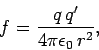(63)

and is directed radially away from the former charge if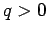, and radially towards it if. Thus, the electric field a distanceaway from a chargehas the magnitude(64)

and is directed radially away from the charge if, and radially towards the charge if. Note that the field is independent of the magnitude of the test charge.

A corollary of the above definition of an electric field is that a stationary chargelocated in an electric fieldexperiences an electrostatic force(65)

whereis the electric field at the location of the charge (excluding the field produced by the charge itself).

Since electrostatic forces are superposable, it follows that electric fields are also superposable. For example, if we have three stationary point charges,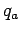,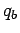, and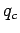, located at three different points in space, then the net electric field which fills space is simply the vector sum of the fields produced by each point charge taken in isolation.Next: Worked Examples Up: Electricity Previous: Coulomb's Law
Richard Fitzpatrick 2007-07-14